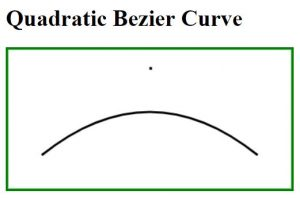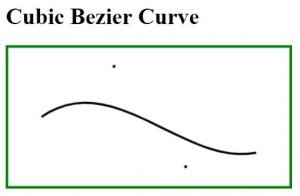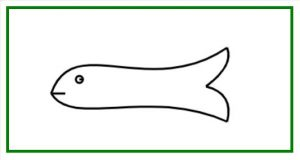# HTML | Canvas Draw Bezier Curve

Curves on HTML canvas can be drawn using arcs, but drawing a complex diagram using arcs is quite a tedious task. In the given circumstance, Bezier curve will be very useful in providing more flexibility in drawing curves. Bezier curves on HTML canvas are drawn using a start point, one or more control point/points and an endpoint. Example: In the case of drawing a landscape, real-world objects, irregular shapes etc.
Bezier Curves can be drawn in two ways:

• Cubic Bezier Curve

Quadratic Bezier Curve: This curve is controlled by one control point.

Syntax:

```moveTo(start_pnt_X, start_pnt_Y);
```

Example: This example create a curve using quadratic bezier curve.

 `  ` `<``html``> ` ` `  `<``head``> ` `    ``<``title``> ` `        ``Quadratic Bezier Curve ` `    `` ` ` ` ` `  `<``body``>  ` `    ``<``h1``>Quadratic Bezier Curve ` `     `  `    ``<``canvas` `id``=``"CanvasOfGeeks"` `width``=``"400"` `height``=``"200"`  `            ``style``=``"border:solid 4px green"``>  ` `     `  `    ``<``script``>  ` `        ``var c = document.getElementById("CanvasOfGeeks"); ` `        ``var context = c.getContext("2d"); ` `         `  `        ``var start_pnt_X = 50; ` `        ``var start_pnt_Y = 150; ` `        ``var cntrl_pnt_X = 200; ` `        ``var cntrl_pnt_Y = 30; ` `        ``var end_pnt_X = 350; ` `        ``var end_pnt_Y = 150; ` `         `  `        ``/* Start new path */ ` `        ``context.beginPath(); ` `        ``context.lineWidth=3;  ` `        ``context.strokeText( ".", cntrl_pnt_X, cntrl_pnt_Y); ` `         `  `        ``/* Starting point of the curve */ ` `        ``context.moveTo(start_pnt_X, start_pnt_Y);  ` `         `  `        ``context.quadraticCurveTo(cntrl_pnt_X,  ` `                    ``cntrl_pnt_Y, end_pnt_X, end_pnt_Y); ` `                     `  `        ``/* drawing line on the canvas */ ` `        ``context.stroke(); ` `    ``  ` `  ` ` `  `                     `

Output:Explanation:

• Pre-requisite: HTML Canvas Basics
• First Line: Reference for canvas object is stored in variable ‘c’ using DOM concept.
Second Line: Without having drawing context of canvas nothing can be drawn on it.

```var c = document.getElementById("CanvasOfGeeks");
var context = c.getContext("2d");
```
• One can change the width of line by overriding the value of “lineWidth” attribute of context object.
`context.lineWidth=3;`
• For putting a dot over the coordinate of control point.You can see the dot in the figure shown above.
`context.strokeText( ".", cntrl_pnt_X, cntrl_pnt_Y);`
• This function is used to draw a curve from the start point mentioned in function.
`context.quadraticCurveTo(cntrl_pnt_X, cntrl_pnt_Y, end_pnt_X, end_pnt_Y);`
• This function is used to move the context.
`context.moveTo(start_pnt_X, start_pnt_Y);`

Note: Please keep the control point within the canvas boundary.

Cubic Bezier Curve: This curve is controlled by two control points.

Syntax:

```moveTo(start_pnt_X, start_pnt_Y);
contex.bezierCurveTo(cntrl_pnt_1_X, cntrl_pnt_1_Y, cntrl_pnt_2_X,
cntrl_pnt_2_Y, end_pnt_X, end_pnt_y);
```

Example: This example create a curve using cubic bezier curve.

 `  ` `<``html``> ` `      `  `<``head``> ` `    ``<``title``> ` `        ``Cubic Bezier Curve ` `    `` ` ` ` ` `  `<``body``>  ` `    ``<``h1``>Cubic Bezier Curve ` `     `  `    ``<``canvas` `id``=``"CanvasOfGeeks"` `width``=``"400"` `height``=``"200"`  `            ``style``=``"border:solid 4px green"``>  ` `     `  `    ``<``script``>  ` `        ``var c = document.getElementById("CanvasOfGeeks");  ` `        ``var context = c.getContext("2d"); ` `         `  `        ``var start_pnt_X = 50; ` `        ``var start_pnt_Y = 100; ` `        ``var cntrl_pnt_1_X = 150; ` `        ``var cntrl_pnt_1_Y = 30; ` `        ``var cntrl_pnt_2_X = 250; ` `        ``var cntrl_pnt_2_Y = 170; ` `        ``var end_pnt_X     = 350; ` `        ``var end_pnt_Y     = 150; ` `         `  `        ``/* Start a new Path */ ` `        ``context.beginPath();      ` `        ``context.lineWidth=3; ` `         `  `        ``/* Representing first control point */ ` `        ``context.strokeText( ".", cntrl_pnt_1_X, cntrl_pnt_1_Y);  ` `         `  `        ``/* Representing second control point */ ` `        ``context.strokeText( ".", cntrl_pnt_2_X, cntrl_pnt_2_Y);  ` `         `  `        ``/* Starting point of the curve */ ` `        ``context.moveTo(start_pnt_X, start_pnt_Y);  ` `        ``context.bezierCurveTo(cntrl_pnt_1_X, cntrl_pnt_1_Y,  ` `            ``cntrl_pnt_2_X, cntrl_pnt_2_Y, end_pnt_X, end_pnt_Y); ` `         `  `        ``/* Drawing line on the canvas  */ ` `        ``context.stroke();  ` `    ``  ` `  ` ` `  `                     `

Output:Example: This example draw a fish using Bezier Curve.
Input:

 ` ` `<``html``>  ` ` `  `<``head``> ` `    ``<``title``> ` `        ``Drawing a fish using Bezier Curve ` `    `` ` ` ` ` `  `<``body``>  ` `    ``<``canvas` `id``=``"CanvasOfGeeks"` `width``=``"400"` `height``=``"200"`  `            ``style``=``"border:solid 4px green"``>  ` `     `  `    ``<``script``>  ` `        ``var c = document.getElementById("CanvasOfGeeks");  ` `        ``var context = c.getContext("2d"); ` `         `  `        ``/* Start a new Path */ ` `        ``context.beginPath(); ` `        ``context.lineWidth=3; ` `             `  `        ``/* Upper curve of the fish, from mouth to tail */ ` `        ``context.moveTo(60, 120); ` `        ``context.bezierCurveTo(90, 30, 200, 130, 310, 55); ` `             `  `        ``/* Lower curve of the fish, from mouth to tail */ ` `        ``context.moveTo(60, 120); ` `        ``context.bezierCurveTo(90, 170, 200, 110, 310, 160); ` `             `  `        ``/* Upper half of tail */ ` `        ``context.moveTo(310, 55); ` `        ``context.quadraticCurveTo(320, 80, 280, 110); ` `             `  `        ``/* lower half of tail */ ` `        ``context.moveTo(310, 160); ` `        ``context.quadraticCurveTo(320, 120, 280, 110); ` `             `  `        ``/* Eye of the fish */ ` `        ``context.moveTo(100, 100); ` `        ``context.arc(100, 100, 5, 0, 2*Math.PI); ` `             `  `        ``/* Mouth of the fish */ ` `        ``context.moveTo(60, 120); ` `        ``context.lineTo(80, 120); ` `        ``context.stroke(); ` `    ``  ` `  ` ` `  `                     `

Output:My Personal Notes arrow_drop_upIf you like GeeksforGeeks and would like to contribute, you can also write an article using contribute.geeksforgeeks.org or mail your article to contribute@geeksforgeeks.org. See your article appearing on the GeeksforGeeks main page and help other Geeks.

Please Improve this article if you find anything incorrect by clicking on the "Improve Article" button below.

Article Tags :
Practice Tags :

Be the First to upvote.

Please write to us at contribute@geeksforgeeks.org to report any issue with the above content.